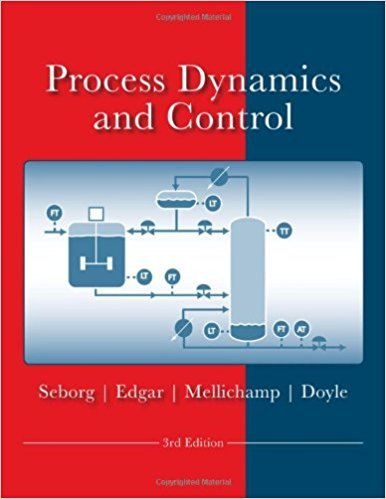×
Get Full Access to Process Dynamics And Control - 3 Edition - Chapter 3 - Problem 3.2
Get Full Access to Process Dynamics And Control - 3 Edition - Chapter 3 - Problem 3.2

×

# Solve the ODE d4y d3y d2y dy - + 16- + 86 d' + 176-dt +ISBN: 9780470128671 148

## Solution for problem 3.2 Chapter 3

Process Dynamics and Control | 3rd Edition

• Textbook Solutions
• 2901 Step-by-step solutions solved by professors and subject experts
• Get 24/7 help from StudySoup virtual teaching assistantsProcess Dynamics and Control | 3rd Edition

4 5 1 385 Reviews
26
2
Problem 3.2

Solve the ODE d4y d3y d2y dy - + 16- + 86 d'? + 176-dt + 105y = 1 dt 4 dr3 r using partial fraction expansion. Note you need to calculate the roots of a fourth-order polynomial in s. All initial conditions on y and its derivatives are zero.

Step-by-Step Solution:
Step 1 of 3

Chemistry Notes Chapter 6: Thermochemistry  Energy- the capacity to do work or transfer heat.  All forms of energy are - Potential - Kinetic  Kinetic energy results from motion and depends on the object’s mass m and its velocity v: KE=1/2 mv^2  Potential Energy is energy possessed by an object by virtue of its position or Stored Energy   Chemical Energy: energy stored in the structural units of substances. The amount of chemical energy depends on the types and arrangement of atoms. Conservation of Energy  Kinetic and Potential energy are interconvertible. Dropping an object converts potential energy to kinetic energy. A chemical reaction that gives off heat converts chemical energy

Step 2 of 3

Step 3 of 3

##### ISBN: 9780470128671

Unlock Textbook Solution